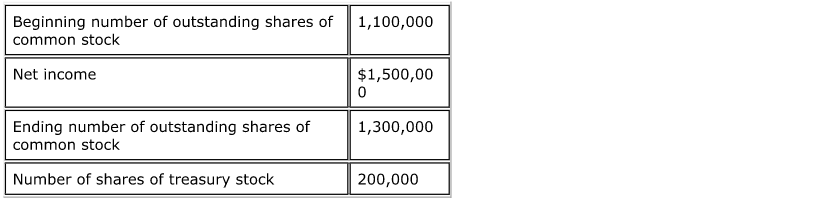# Problem: Consider the following: What is the earnings per share? A) $1.50 B)$1.25 C) $1.20 D)$1.15

###### Problem Details

Consider the following:

What is the earnings per share?

A) $1.50 B)$1.25

C) $1.20 D)$1.15